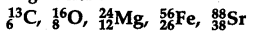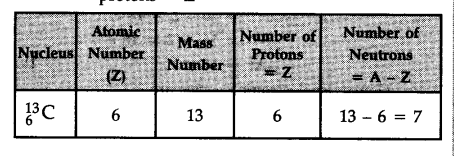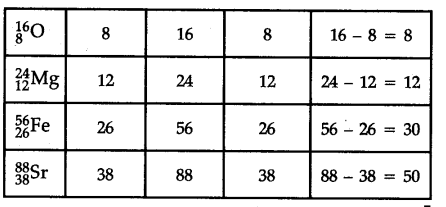# How many neutrons and protons are there in the following nuclei?

How many neutrons and protons are there in the following nuclei ?The digit written as subscript represents the atomic numbers (Z) and that as superscript represents the mass number (A) of the element.
Number of neutrons = A - Z and number of protons = Z